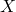In a previous post I made a reference to the estimation of equilibrium effects in the context of a spatial lag model. This is a question which has received surprisingly little attention given that the standard approach to interpreting parameter estimates is generally inapplicable in this setting. The problem is that in a spatial lag model, the effect of any given variable depends on the structure of geographic relationships in the underlying data. To the extent that these relationships vary across observations, the relationship between some independent variableand some dependent variablevaries across observations as well.

This can be seen by expressing the conventional spatial lag modelin terms of the following reduced form representation:whereis anresponse vector,is anidentity matrix,is a measure of spatial autocorrelation,is anspatial weights matrix depicting the relationship between observations,is anmatrix of covariates,is avector of parameter estimates, andis anvector of errors. In this context, the expected effect of a one-unit change in any given covariateis equal to. Thus, the standard interpretation ofonly holds in the absence of spatial autocorrelation (i.e. when). Moreover, instead of having a single estimated effect expressed solely in terms of, we have a system of effects expressed in terms ofmatrix depicting the way in which changes inin observationinfluence the value ofin observation.

Equilibrium effects are simply the set of effects which fall along the diagonal of this larger system. The reason why they are referred to as “equilibrium” effects is because they reflect the relationship betweenandin observation, net of any feedback within the system. Roughly speaking, equilibrium effects can be thought of as unit-specific estimates of. LeSage and Pace (2009) differentiate these types of “direct impacts” from both the “indirect impacts” depicted by the off-diagonal entries of, as well as the “total impacts” represented by the row and column sums of. Whether we consider rows or columns depends on whether we are interested in the impacts coming to or from an observation, respectively.

What are we to do with all of this information? One approach is to consider the distribution of equilibrium effects. This is a useful tool for displaying the way in which a given relationship varies across observations. Note, however, that insofar as the parameteris simply a scale factor, comparing the distribution of effects across variables is largely uninformative due to the fact that the shape of these distributions is driven by relationships contained in the matrixwhich is, by definition, constant across the set of independent variables. Another approach is to construct summary measures of the direct, indirect, and total impacts. This is discussed at length in chapter 2 of the LeSage and Pace text mentioned above. These measures can be implemented in R using the impacts routine included as part of the spdep library.

Discussion of these issues has largely been limited to the fields of economics and, to a lesser extent, political science. This is somewhat problematic given the non-trivial number of articles in sociology which have used the spatial lag model to try and capture processes such as diffusion. To be fair, a parameter estimate produced using a spatial lag model is probably going to be pretty close to the average equilibrium effect. So while existing articles may be technically wrong in their interpretation of the effects associated with a given set of covariates, it is unlikely that their substantive stories are somehow radically off-base.